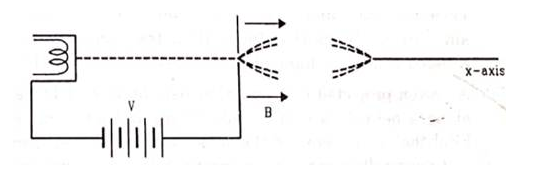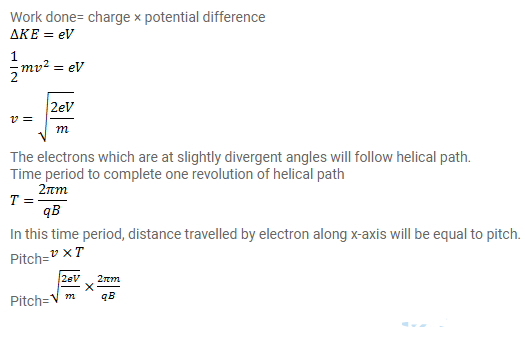# Electrons are emitted with negligible speed from an electron gun

Question:

Electrons are emitted with negligible speed from an electron gun are accelerated through a potential difference $V$ along the $X$-axis. These electrons emerge from a narrow hole into a uniform magnetic field B directed along this axis. However, some of the electrons emerging from the hole make slightly divergent angles as shown in figure. Show that these paraxial electrons are focused on the $X$-axis at a distance

$\sqrt{\frac{8 \pi^{2} m V}{e B^{2}}}$Solution: